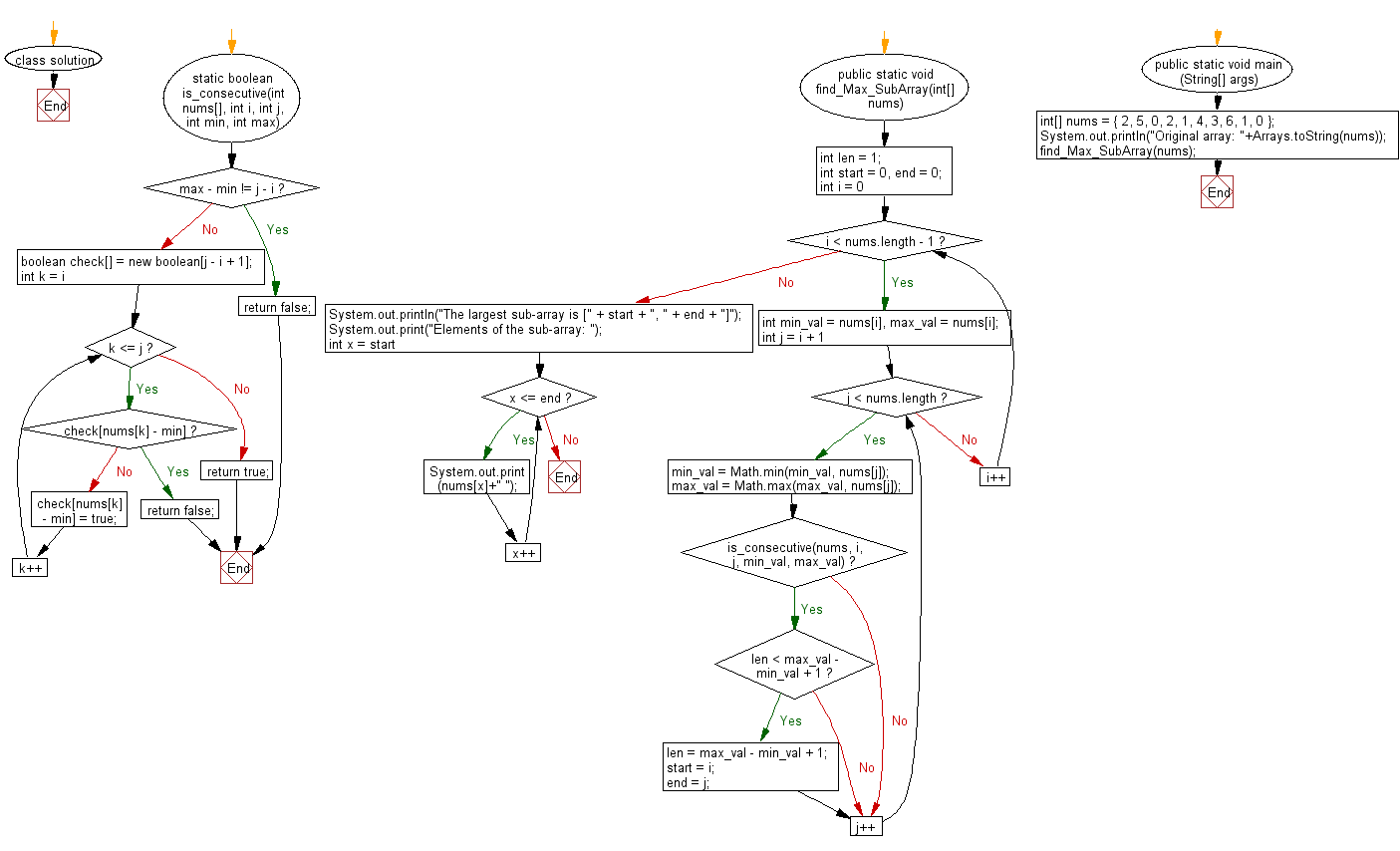﻿ Java: Check if a sub-array is formed by consecutive integers# Java Array Exercises: Check if a sub-array is formed by consecutive integers from a given array of integers

## Java Array: Exercise-57 with Solution

Write a Java program to check if a sub-array is formed by consecutive integers from a given array of integers.

Example:
Input :
nums = { 2, 5, 0, 2, 1, 4, 3, 6, 1, 0 }
Output:
The largest sub-array is [1, 7]
Elements of the sub-array: 5 0 2 1 4 3 6

Sample Solution:

Java Code:

``````import java.lang.Math;
import java.util.Arrays;
class solution
{
static boolean is_consecutive(int nums[], int i, int j, int min, int max)
{
if (max - min != j - i) {
return false;
}

boolean check[] = new boolean[j - i + 1];

for (int k = i; k <= j; k++)
{
if (check[nums[k] - min]) {
return false;
}

check[nums[k] - min] = true;
}

return true;
}

public static void find_Max_SubArray(int[] nums)
{
int len = 1;
int start = 0, end = 0;

for (int i = 0; i < nums.length - 1; i++)
{
int min_val = nums[i], max_val = nums[i];

for (int j = i + 1; j < nums.length; j++)
{
min_val = Math.min(min_val, nums[j]);
max_val = Math.max(max_val, nums[j]);

if (is_consecutive(nums, i, j, min_val, max_val))
{
if (len < max_val - min_val + 1)
{
len = max_val - min_val + 1;
start = i;
end = j;
}
}
}
}

System.out.println("The largest sub-array is [" + start + ", "
+ end + "]");
System.out.print("Elements of the sub-array: ");
for (int x = start; x <= end; x++)
{

System.out.print(nums[x]+" ");
}
}

public static void main (String[] args)
{
int[] nums = { 2, 5, 0, 2, 1, 4, 3, 6, 1, 0 };
System.out.println("Original array: "+Arrays.toString(nums));
find_Max_SubArray(nums);
}
}
```
```

Sample Output:

```
Original array: [2, 5, 0, 2, 1, 4, 3, 6, 1, 0]
The largest sub-array is [1, 7]
Elements of the sub-array: 5 0 2 1 4 3 6
```

Flowchart:Java Code Editor:

Improve this sample solution and post your code through Disqus

What is the difficulty level of this exercise?

Test your Programming skills with w3resource's quiz.

﻿

## Java: Tips of the Day

countOccurrences

Counts the occurrences of a value in an array.

Use Arrays.stream().filter().count() to count total number of values that equals the specified value.

```public static long countOccurrences(int[] numbers, int value) {
return Arrays.stream(numbers)
.filter(number -> number == value)
.count();
}
```

Ref: https://bit.ly/3kCAgLb